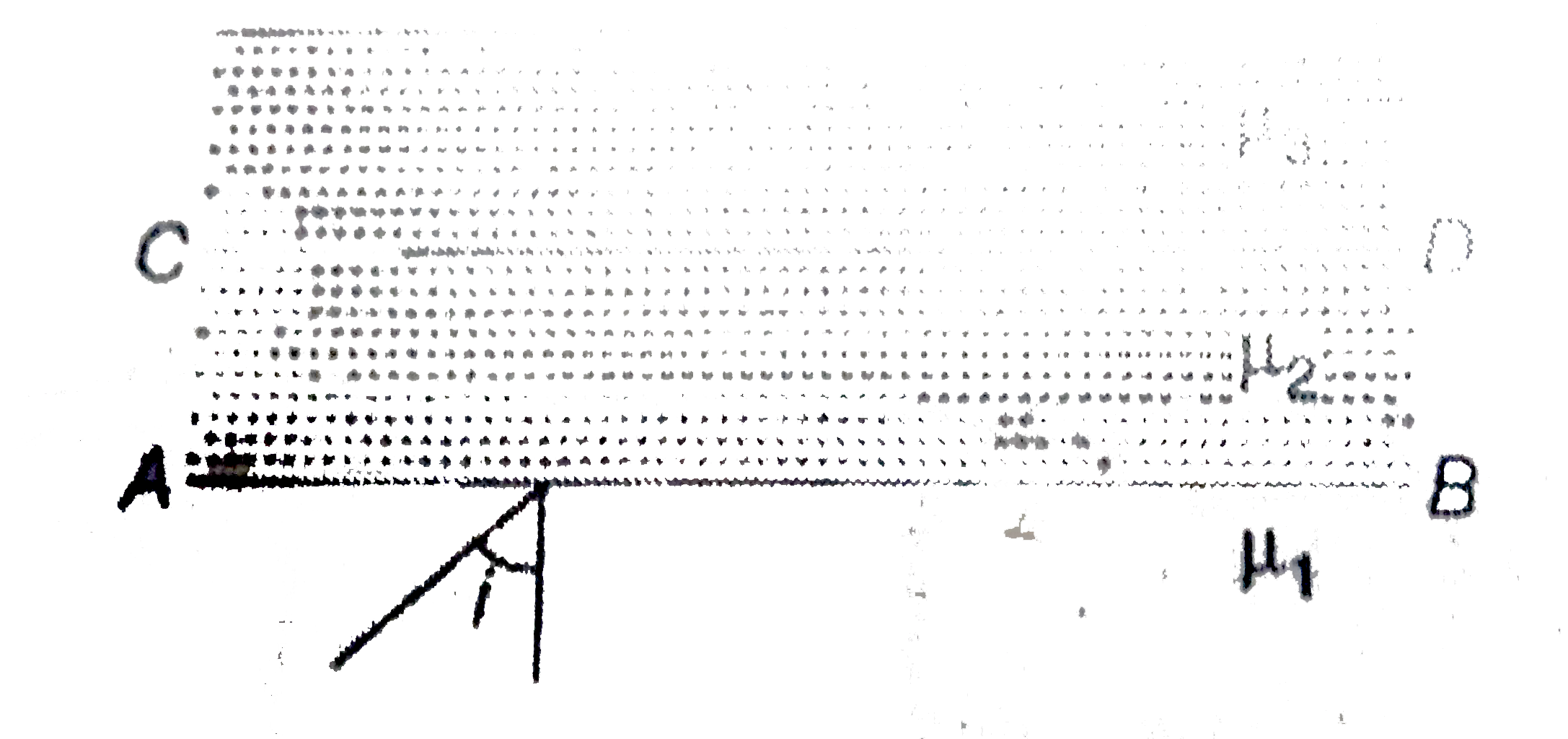# In the figure shown, mu_(1) gt mu_(2) gt mu_(3). What are the limits of angle i so that it is neither get total internal reflection at AB nor at CD

21 views
in Physics
In the figure shown, mu_(1) gt mu_(2) gt mu_(3). What are the limits of angle i so that it is neither get total internal reflection at AB nor at CD ?A. sin i lt (mu_(2))/((mu_(2))
B. sin i lt (mu_(3))/((mu_(1))
C. sin i lt (mu_(3))/((mu_(2))
D. None of these

by (120k points)